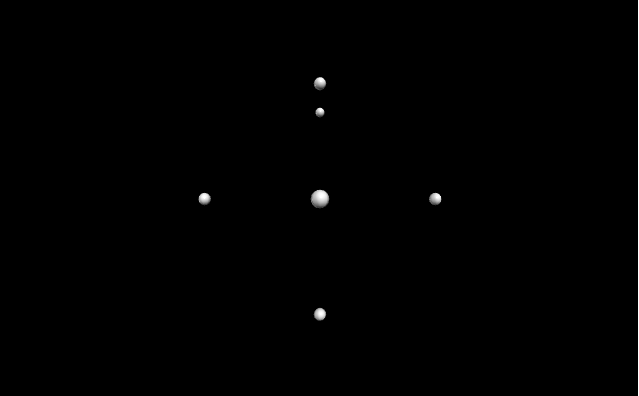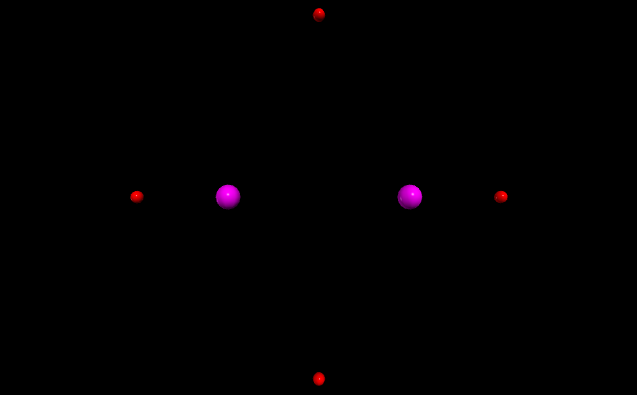GeeksforGeeks App
Open AppBrowser
Continue

# Making points in VPython

`VPython` makes it easy to create navigable 3D displays and animations, even for those with limited programming experience. Because it is based on Python, it also has much to offer for experienced programmers and researchers. `VPython` allows users to create objects such as spheres and cones in 3D space and displays these objects in a window. This makes it easy to create simple visualizations, allowing programmers to focus more on the computational aspect of their programs. The simplicity of `VPython` has made it a tool for the illustration of simple physics, especially in the educational environment.

Installation :

`pip install vpython`

A point in geometry is a location. We can generate points in `VPython` using the `points()` method.

## points()

Syntax : points(parameters)

Parameters :

• pos : It is the position of the points. Assign a list of vectors containing 3 values, example pos = [vector(-1, 0, 0), vector(1, 0, 0)]
• color : It is the color of the points. Assign a vector containing 3 values, example color = vector(1, 1, 1) will give the color white
• radius : It is the radius of the points. Assign a floating value, the default radius is 2.5, example radius = 5
• size : It is the size of the cylinder. Assign a vector containing 3 values representing the length, height and width respectively, example size = vector(1, 1, 1)

All the parameters are optional.

Without any parameters, the `points()` method will not display anything by default.

Example 1 :Points using the parameter pos.

 `# import the module``from` `vpython ``import` `*` `points(pos ``=``[vector(``-``1``, ``0``, ``0``), ``            ``vector(``1``, ``0``, ``0``),``            ``vector(``0``, ``1``, ``0``),``            ``vector(``0``, ``-``1``, ``0``),``            ``vector(``0``, ``0``, ``1``),``            ``vector(``0``, ``1``, ``-``1``)])`

Output :Example 2 :Points using the parameters color and radius.

 `# import the module``from` `vpython ``import` `*`` ` `# first set of points``points(pos ``=``[vector(``-``1``, ``0``, ``0``), ``            ``vector(``1``, ``0``, ``0``),``            ``vector(``0``, ``1``, ``0``),``            ``vector(``0``, ``-``1``, ``0``)],``       ``color ``=` `vector(``1``, ``0``, ``0``),``       ``radius ``=` `10``)`` ` `# second set of points``points(pos ``=``[vector(``-``0.5``, ``0``, ``0``), ``            ``vector(``0.5``, ``0``, ``0``)],``       ``color ``=` `vector(``1``, ``0``, ``1``),``       ``radius ``=` `20``)`

Output :My Personal Notes arrow_drop_up